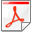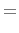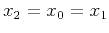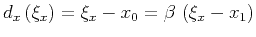The time and space formulation of azimuth moveout# APPENDIX B: DERIVING THE AMO APERTURE

amosym
Figure 5.
Reflection from the ellipsoid of a prestack migration impulse response (a scheme). Top: Map view. Bottom: Section of the ellipsoid with the plane drawn through the central line and the reflection point.This appendix describes the derivation of the main formulas for the aperture evaluation that follow from the Fermat principle (18). In order to avoid the algebraic complications of (18), we simplify the problem by taking into account the cylindrical symmetry of the ellipsoidal reflector (15).

Consider a plane drawn through the reflection point and the central line of the ellipsoid (the axis of the cylindrical symmetry). This plane has to contain the central (normally reflected) ray from the reflector. This conclusion follows from the fact that all the normal reflections emerge at the central line because of the cylindrical symmetry, as shown in Figure B-1. The intersection of the 3-D reflector and the plane is the 2-D ellipse(28)

The connection between the emergence point of the normal rayand thecoordinate of the reflection pointcan be derived from the relationship evident in Figure B-1, as follows:(29)

Equation (B-2) allows us to evaluatein terms ofand get (19). The emergence point of the normal raycorresponds to the midpoint on an imaginary zero-offset section ( with a coincident source and receiver). Therefore, the location of this point is determined for given input and output midpoints in accordance with expression (7).

Obviously, the reflection point has to be inside the ellipse (B-1). Therefore, its projection obeys the inequality(30)

As follows from (B-3), (B-2), and (16),(31)

Inequality (B-4) is the known aperture limitation of the DMO operator (2) found by Deregowski and Rocca (1981). The equality in (B-4) is achieved when the reflection point is on the surface, where the reflector dip increases to 90 degrees.

Now the only unknown left in our problem is the-coordinate of the reflection point. To find this unknown, we substitute (19) into (17), choosing the convenient parameterization(32)

where, and(Figure B-1). The two-point traveltime function in (17) transforms to the form(33)

Applying the second equation from (18), we get a simple linear equation for, which has the explicit solution (20). From (19) and (20) one can find the reflection point location for given midpoint and offset. To find the limits of possible output midpoint locations, we constrain the reflection point to be inside the ellipsoid (15) similarly to the way we did in two dimensions when deriving (B-4). First, let's consider the case of(the output midpointis on the line drawn throughin the direction of the input azimuth). In this case, combining expression (20) and inequality (21) produces(34)

For any azimuth rotation angleless than 90 degrees, the limitation (B-7) is smaller than that of the DMO operator (B-4). The difference increases with the decrease of the azimuth rotation, since the AMO aperture section on the linemonotonously shrinks to a pointwhenapproaches zero. To extend this conclusion to the whole 3-D aperture, we can find the contour of the aperture by putting the reflection point at the edge of the ellipsoid (15), as follows:(35)

and solving (20) for. The aperture contour can then be defined by the system of parametric expressions(36)(37)

where(38), andis defined by (B-8).The time and space formulation of azimuth moveout